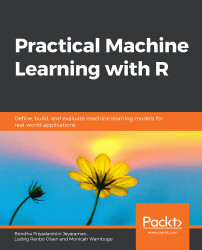•#### Practical Machine Learning with R#### Overview of this book

With huge amounts of data being generated every moment, businesses need applications that apply complex mathematical calculations to data repeatedly and at speed. With machine learning techniques and R, you can easily develop these kinds of applications in an efficient way. Practical Machine Learning with R begins by helping you grasp the basics of machine learning methods, while also highlighting how and why they work. You will understand how to get these algorithms to work in practice, rather than focusing on mathematical derivations. As you progress from one chapter to another, you will gain hands-on experience of building a machine learning solution in R. Next, using R packages such as rpart, random forest, and multiple imputation by chained equations (MICE), you will learn to implement algorithms including neural net classifier, decision trees, and linear and non-linear regression. As you progress through the book, you’ll delve into various machine learning techniques for both supervised and unsupervised learning approaches. In addition to this, you’ll gain insights into partitioning the datasets and mechanisms to evaluate the results from each model and be able to compare them. By the end of this book, you will have gained expertise in solving your business problems, starting by forming a good problem statement, selecting the most appropriate model to solve your problem, and then ensuring that you do not overtrain it.Free Chapter
An Introduction to Machine LearningData Cleaning and Pre-processingFeature EngineeringIntroduction to neuralnet and Evaluation MethodsLinear and Logistic Regression ModelsUnsupervised LearningAppendix## Linear Regression

When performing linear regression, we are trying to find linear relationships between variables. Suppose we have a cat shelter and want to know how many extra cans of cat food we need to buy after receiving new cats. A simple approach would be to find the average number of cans a cat eats per day (z) and multiply it by the number of new cats (x). This is a linear relationship: if the number of cats increases by x, make sure to buy x times z cans of cat food. Of course, other variables might affect how much a new cat eats, such as age, breed, and weight at birth. We could possibly make a better linear model by adding these as predictors.

Imagine we had measured the amount of food eaten by 85 cats, along with their weight at birth (in grams). For budgeting reasons, we wish to predict the amount of food (number of cans) a newborn cat will eat per day when it grows up. We can plot these variables against each other, as shown in Figure 5.2: